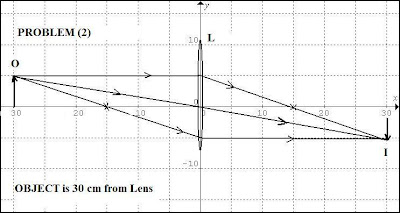## Friday, June 17, 2011

### Solutions to Lens Problems (Part 18)We now look at the lens problems from Part 18 and their solutions. To recap, the problems were:

1) Using the experimental information and the lens diagram graphic as a guide, construct a lens diagram and thereby find the height of the image formed in the student's experiment.

2) By a similar graphical construction, find the position of the image formed by a converging lens (f = 15 cm) when:

a) the object is 60 cm from the lens

b) the object is 30 cm from the lens

3) A lamp and a screen are 160 cm apart. What should the focal length of the lens be in order to produce a real image of the lamp three times as large as the source?

(Hint: we can use the proportion: h'/h = s'/s to find image or object dimensions using image and object distances)

Solutions:

1- The solution to Problem (1) is given in the top diagram (identified as Problem 1). As may be seen, the object is taken as 5 cm in height, and the image is smaller and inverted.

2- The solutions to Problem (2) are given in the respective diagrams for object distances of s = 30 cm and s = 60 cm. The image sizes can each be worked out from the scales shown.

3- We have: h'/h = s'/s and the image-screen dist. = 160 cm so that:

s + s' = 160 cm

Then:

since we need: h' = (s'/s)h = 3h (since s' = 3s) then:

s + 3s = 160 cm

4s = 160 cm

or s = 40 cm

therefore, s' = 160 cm - 40 cm = 120 cm.

Then f can be found from thin lens eqn. given s' = 120 cm and s = 40 cm

f = (s s')/ (s + s') = (120 cm)(40 cm)/ (120 cm + 40 cm)

f = 4800 cm/ 160 = 30 cm